ATM
¤
April 11, 2017
Complex Variables: Mapping Diagrams for
Visualizing Complex Arithmetic and Functions
Dynamically with GeoGebra

 Part I Mapping Diagrams for Real Functions Complex Arithmetic Part II Complex Functions

Martin Flashman
Professor of Mathematics
Humboldt State University

http://flashman.neocities.org/Presentations/MD.Reed.CV.9_19_16.html

Abstract: Mapping diagrams provide a valuable tool for visualizing complex variable arithmetic and functions. Using GeoGebra, I will demonstrate with dynamic diagrams important properties of complex arithmetic and functions. Examples will include complex multiplication and division, linear and linear fractional functions. No prior knowledge of complex arithmetic and functions will be presumed.
Background and References to other work on Mapping Diagrams for real variables ¤

1.1.Background: Mapping Diagrams for Real Functions . ¤

1.1.1 What is a mapping diagram?
Introduction and simple examples from the past: Napiers LogarithmSee: http://www.maa.org/press/periodicals/convergence/logarithms-the-early-history-of-a-familiar-function-john-napier-introduces-logarithms

Logarithms: The Early History of a Familiar Function - John Napier Introduces Logarithms  by Kathleen M. Clark (The Florida State University) and Clemency Montelle (University of Canterbury) ¤1.1.2 Real Functions. ¤
Understanding Real Functions: Tables, Mapping Diagrams, and Graphs

1.2. Real Linear Functions. ¤
Real Linear functions are the key to understanding calculus.
Linear functions are traditionally expressed by an equation like :
$f(x)= mx + b$.
Mapping diagrams for real linear functions have one simple unifying feature- the focus point, determined by the numbers
$m$ and $b$, denoted here by $[m,b]$
.
Mapping Diagrams and Graphs of Real Linear Functions
Visualizing real linear functions using mapping diagrams and graphs.
This is a Java Applet created using GeoGebra from www.geogebra.org - it looks like you don't have Java installed, please go to www.java.com
Notice how points on the graph pair with arrows and points on the mapping diagram.

2.1 Complex Numbers:     $\mathbb{C}$   ¤
Definition: The "number" $i$:

To solve the equation $x^2 +1 =0$ we create a symbol $i$ and declare that $i^2 = -1$ so $i$ solves the equation  and we write $$i = \sqrt{ -1 }.$$

Definitions: A complex number $z$ is a number that can be expressed in the form $z =a +bi$ where $a$ and $b$ are real numbers.

If
$z =a +bi$ then $a$ is called the real part of $z$ and $bi$ (and sometimes $b$) is called the imaginary part of $z$.
Examples: $3 + 2i$ and $-2 - i$.

 2.2 Complex Geometry- The Complex Plane Complex numbers can be identified with points in a cartesian plane by having $a +bi$ identified with the point with coordinates $(a,b)$ or with position vectors by identifying $a+bi$ with the vector $$. With this identification i is identified with the point (0,1) and -i is identified with (0,-1). 2.2.1 Complex Number Norm (Magnitude): The norm of z is defined by |z| = |a+bi| = \sqrt{ a^2 +b^2} 2.2.2 Polar Representation of z: ¤ Using trigonometry we have the identification:$$z = |z| \cos( \theta) + |z| \sin(\theta) i = |z| [\cos( \theta) + \sin(\theta) i] = |z| cis( \theta) $$where a = \cos( \theta ), b = \sin(\theta).$$z$$is sometimes represented as an ordered pair$$(|z|, \theta)$$. The angle \theta determined by z can be measured in degrees or radians and restricted to be in a specific interval. For example \theta \in [0,2 \pi) or \theta \in (-\pi, \pi]. Thus the angle can be considered a function of z, called the argument of z: Arg(z) =\theta.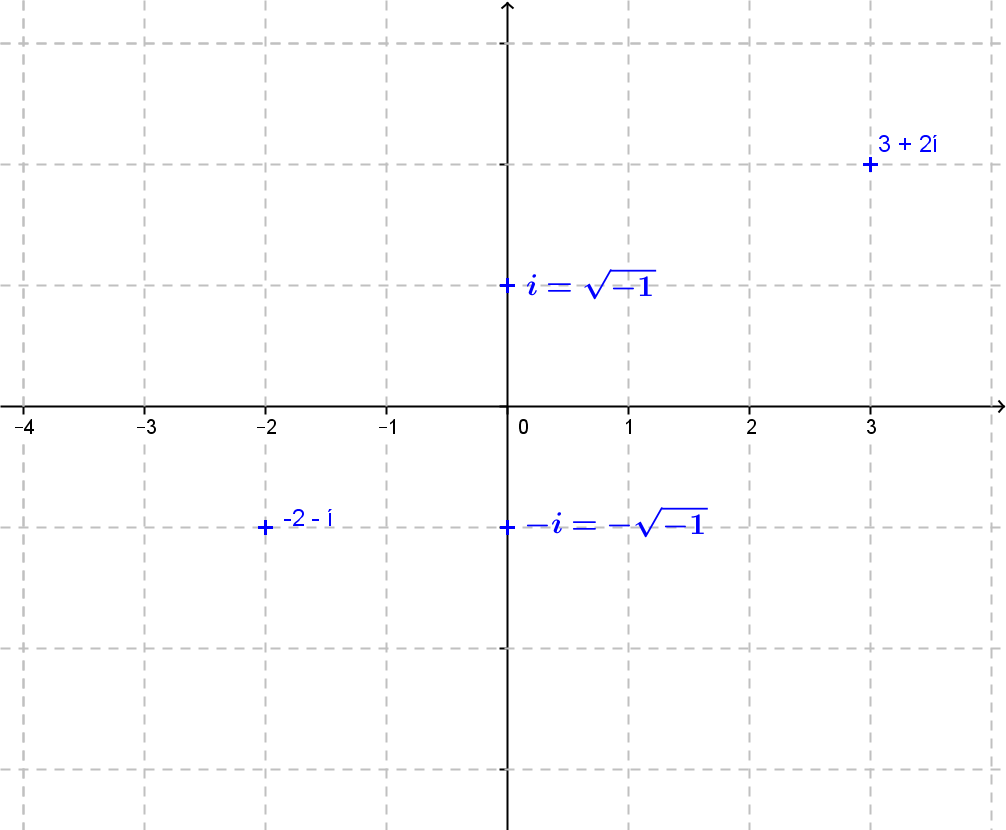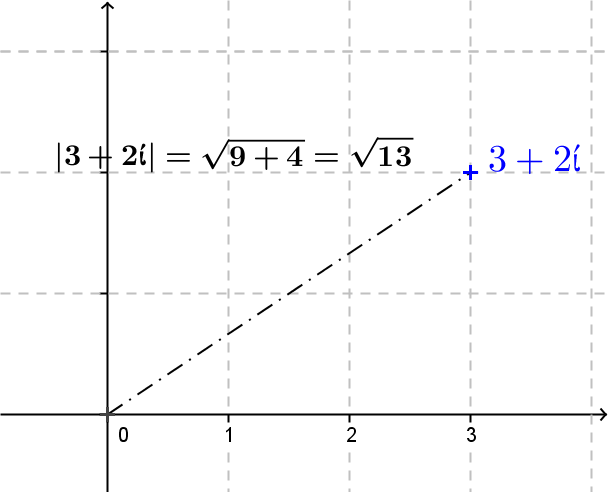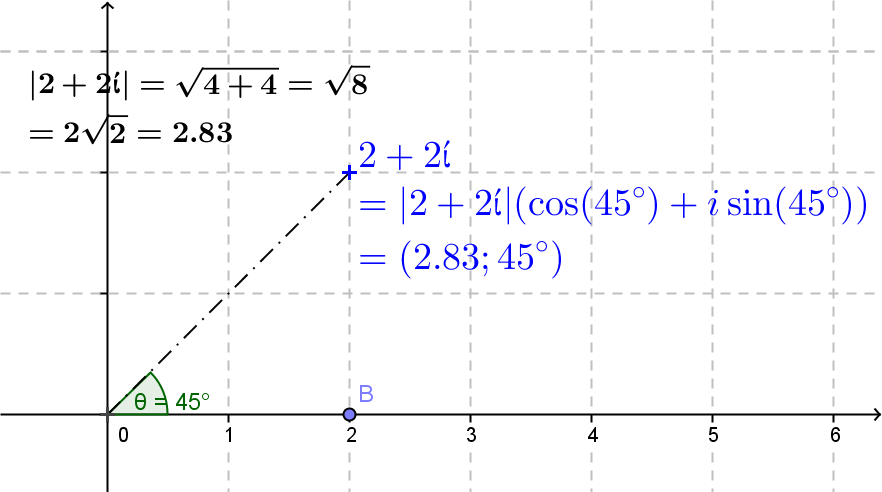2.2.3 Exponential Representation of z: ¤ Consider the Taylor Series for the functions:$$e^x = 1 + x + \frac{x^2}2 +\frac{x^3}{3!} + \frac{x^4}{4!} + \frac{x^5}{5!} + \frac{x^6}{6!} + \frac{x^7}{7!}  ...\cos(x) = 1 - \frac{x^2}2 + \frac{x^4}{4!} - \frac{x^6}{6!} + ...\sin(x) =  x - \frac{x^3}{3!} +  \frac{x^5}{5!}  -  \frac{x^7}{7!}  ...$$Then one can show with the series using \theta in radian measure:$$e^{i\theta}= \cos(\theta) + \sin(\theta)i = cis (\theta)$$and thus$$z =|z|e^{i \theta}$$. Note: When \theta =\pi this equation demonstrates that e^{\pi i} = \cos(\pi) + sin(\pi)i = -1. 2.3 Complex Arithmetic  2.3.1 Complex Addition: z_1+z_2 ¤ If z_1 = a_1 + b_1i and z_2 = a_2 + b_2i then$$z_1+z_2 =a_1 + a_2 + (b_1+ b_2) i $$Example: If z_1 = 2+3i; z_2= 1 - i then z_1+z_2= (2+1) + (3-1) i = 3 + 2i . The addition can be thought of as vector addition - with separate addition of the real and imaginary parts. Addition can be visualized in the complex plane by using parallelograms.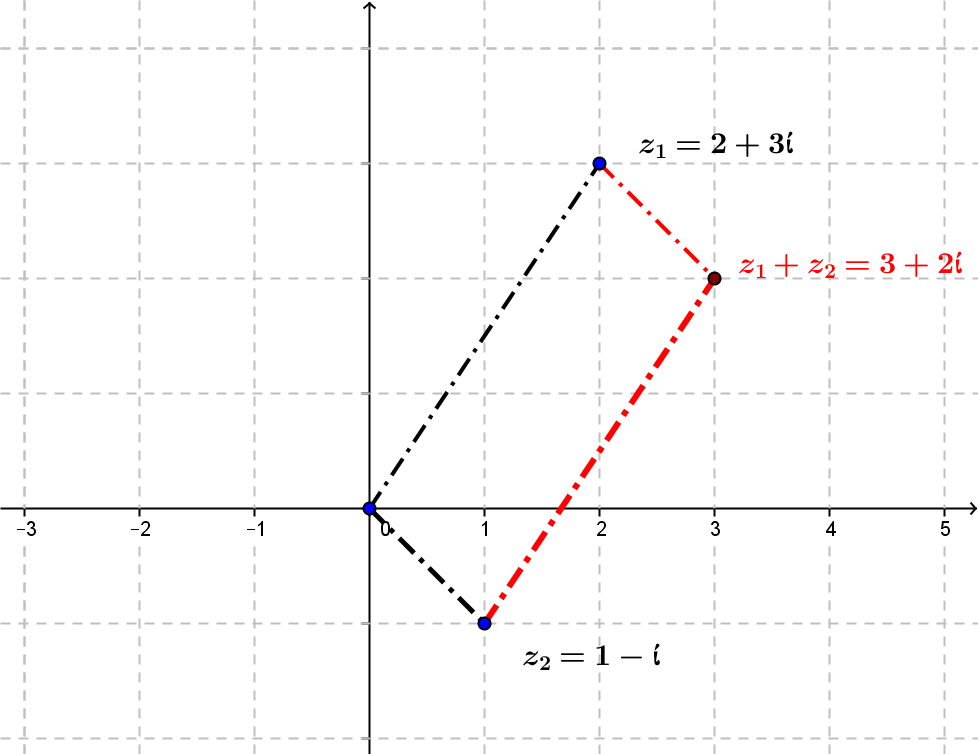2.3.2 Complex Multiplication: z_1 \cdot z_2 ¤  Algebraically:If z_1 = 2+3i; z_2= 1 - i then z_1 \cdot z_2= (2+3i) \cdot (1-i) = 2 \cdot 1 - 3\cdot i^2 + 3i \cdot 1 +2 \cdot (-i) = (2+3) +(3- 2)i = 5+i. It is possible understand complex multiplication geometrically using the polar or the exponential representation (in radian measure): z_1=|z_1| [\cos( \theta_1) + \sin(\theta_1) i] = |z_1|e^{i\theta_1} ;\ z_2=\cdot |z_1| [\cos( \theta_2) + \sin(\theta_2) i] = |z_2|e^{i\theta_2} z_1 \cdot z_2= |z_1| [\cos( \theta_1) + \sin(\theta_1) i] \cdot |z_1| [\cos( \theta_2) + \sin(\theta_2) i]   = |z_1|\cdot |z_1| [\cos( \theta_1) + \sin(\theta_1) i] \cdot [\cos( \theta_2) + \sin(\theta_2) i]  = |z_1|\cdot |z_1| [\cos( \theta_1)\cos( \theta_2) - \sin(\theta_1)\sin(\theta_2) + (\sin(\theta_1)\cos( \theta_2) + \sin(\theta_2) \cos(\theta_1) )i]  =|z_1|\cdot |z_1| [\cos( \theta_1 + \theta_2) + \sin(\theta_1 +\theta_2) i]  =|z_1|\cdot |z_1| cis (\theta_1 +\theta_2)  or more simply using \theta in radian measure: z_1 \cdot z_2= |z_1|e^{i\theta_1}\cdot |z_2|e^{i\theta_2}  =|z_1|\cdot |z_1| e^{(\theta_1 +\theta_2)i}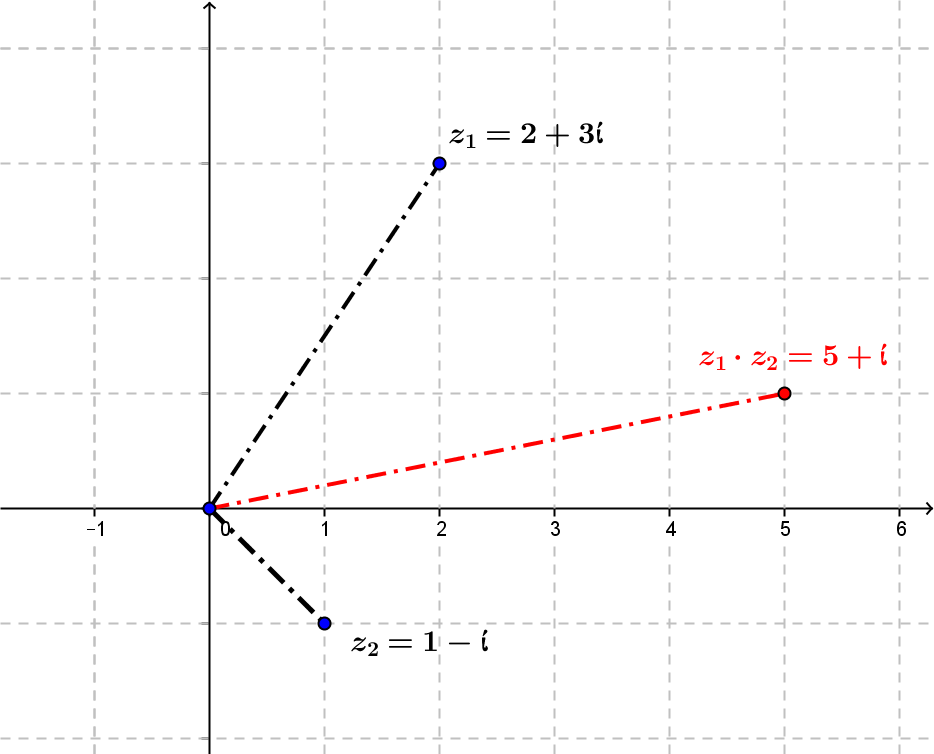Example: If z_1 = \sqrt 2\ cis(45°); z_2= 2\ cis(30°) then z_1\cdot z_2= 2\sqrt 2\ cis (75 °) .¤ 2.3.3 Complex Inverses:  \frac1z, z \ne 0 ¤  Example: Express \frac 1{2+3i} in the standard form of a + bi. Solution: \frac 1{2+i} =\frac 1{2+i} \cdot \frac {2-i}{2-i} = \frac{2-i}{4+1} = \frac 2{5} - \frac 3{5}i. Fact: If a^2+b^2 \ne 0 then \frac1{a+bi} = \frac a{a^2 + b^2} - \frac b{a^2 + b^2}i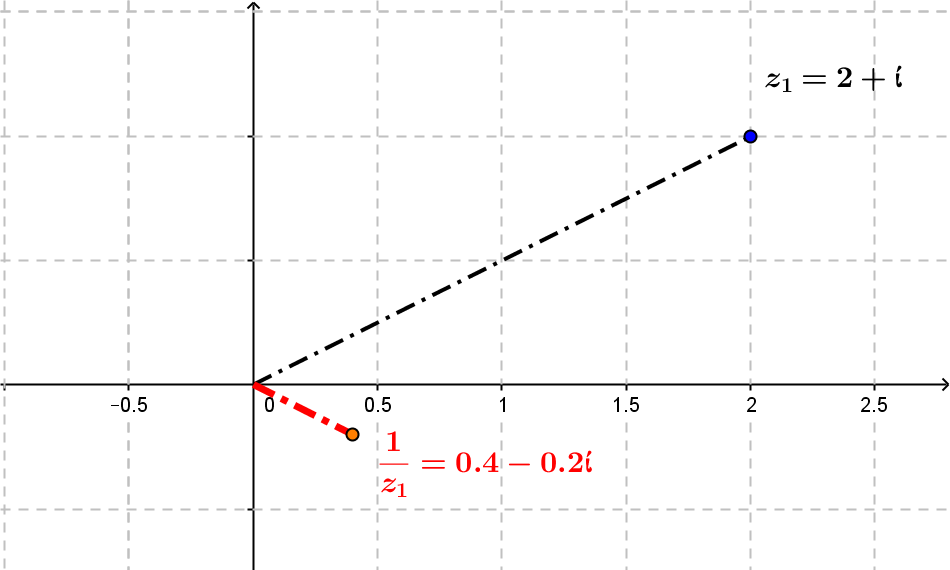2.3.4 Geometry for  \frac1z, z \ne 0 Geometric understanding of  \frac1z, z \ne 0 comes best by using the polar or exponential representation and the multiplicative inverse property: z \cdot\frac1z = 1. If we let z=|z [\cos( \theta) + \sin(\theta) i] ;\ z_1=\frac1z= |z_1| [\cos( \theta_1) + \sin(\theta_1) i]  then$$z \cdot z_1= |z|\cdot |z_1| [\cos( \theta + \theta_1) + \sin(\theta +\theta_1) i] =|z|\cdot |z_1| cis (\theta +\theta_1) = 1=1\ cis(0^{\circ} )   then $|z_1| = \frac 1{|z|}$ and $\theta_1 =-\theta$.

That is, if $z=|z|\ cis(\theta) = |z|e^{i\theta}$ then $\frac1z = \frac1{|z|} \ cis(-\theta) = \frac1{|z|} e^{-i\theta}$